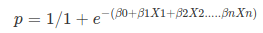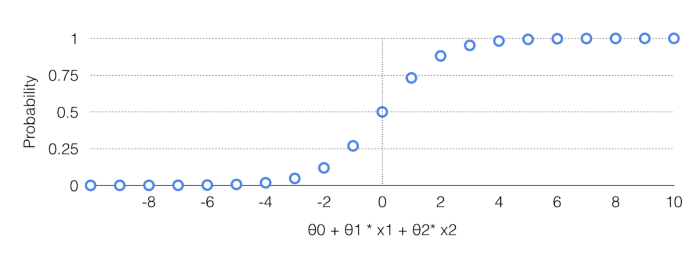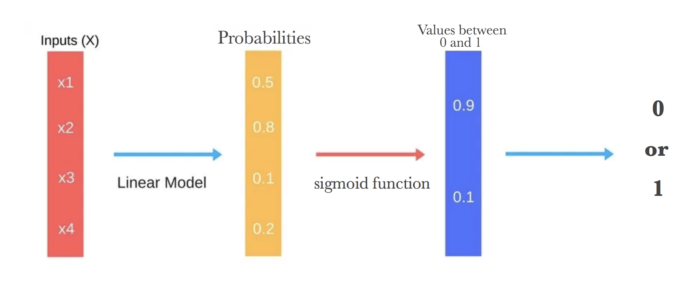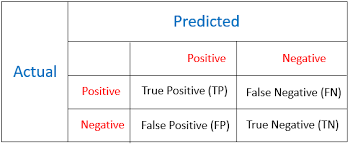top of page
Search
• Nagesh Singh Chauhan

# Real world implementation of Logistic Regression

### A step by step approach to build both Binary and Multiclass Logistic Regression modelsCredits: https://www.appgate.com/blog/

Classification techniques are an essential part of machine learning and data mining applications. Approximately 70% of problems in Data Science are classification problems. A popular classification technique to predict binomial outcomes (y = 0 or 1) is called Logistic Regression. Logistic regression predicts categorical outcomes (binomial/multinomial values of y), whereas linear Regression is good for predicting continuous-valued outcomes (such as the weight of a person in kg, the amount of rainfall in cm).

I have divided this article into 3 parts. In the first part we’ll take a look at some of the important concepts of Logistic Regression, In the second part we’ll build a Binary classifier and in the third part, we’ll build a Multiclass classifier.

Table of Content:

• Logistic Regression

• Maximum Likelihood Estimation Vs. Ordinary Least Square Method

• Sigmoid Function

• Logistic Regression assumptions

• Binary Logistic Regression model building in Scikit-learn

• Model Evaluation using Confusion Matrix

• Multiclass Logistic Regression model building in Scikit-learn

• Model Evaluation using Confusion Matrix

# Logistic Regression

Logistic regression is a statistical method for predicting binary classes. The outcome or target variable is binary in nature. For example, it can be used for cancer detection problems. It computes the probability of an event occurrence.

It is a special case of linear regression where the target variable is categorical in nature. It uses a log of odds as the dependent variable. Logistic Regression predicts the probability of occurrence of a binary event utilizing a logit function.

Linear Regression Equation:Where, y is dependent variable and x1, x2 … and Xn are explanatory variables.

Sigmoid Function:Apply Sigmoid function on linear regression:Properties of Logistic Regression:

• The dependent variable in logistic regression follows Bernoulli Distribution.

• Estimation is done through maximum likelihood.

• No R Square, Model fitness is calculated through Concordance, KS-Statistics.

# Maximum Likelihood Estimation

The MLE is a “likelihood” maximization method, while OLS is a distance-minimizing approximation method. Maximizing the likelihood function determines the parameters that are most likely to produce the observed data. From a statistical point of view, MLE sets the mean and variance as parameters in determining the specific parametric values for a given model. This set of parameters can be used for predicting the data needed in a normal distribution.

# Sigmoid FunctionThe sigmoid function also called the logistic function gives an ‘S’ shaped curve that can take any real-valued number and map it into a value between 0 and 1. If the curve goes to positive infinity, y predicted will become 1, and if the curve goes to negative infinity, y predicted will become 0. If the output of the sigmoid function is more than 0.5, we can classify the outcome as 1 or YES, and if it is less than 0.5, we can classify it like 0 or NO. If the output is 0.75, we can say in terms of probability as: There is a 75 percent chance that patient will suffer from cancer.The sigmoid curve has a finite limit of:

‘0’ as x approaches −∞

‘1’ as x approaches +∞

The output of sigmoid function when x=0 is 0.5

Thus, if the output is more tan 0.5, we can classify the outcome as 1 (or YES) and if it is less than 0.5, we can classify it as 0(or NO).

For example: If the output is 0.65, we can say in terms of probability as:

“There is a 65 percent chance that your favorite cricket team is going to win today ”.

# Logistic Regression Assumptions

• Binary logistic regression requires the dependent variable to be binary.

• For a binary regression, the factor level 1 of the dependent variable should represent the desired outcome.

• Only meaningful variables should be included.

• The independent variables should be independent of each other. That is, the model should have little or no multicollinearity.

• The independent variables are linearly related to the log odds.

• Logistic regression requires quite large sample sizes.

# Binary Logistic Regression model building in Scikit learnA Binary logistic regression (often referred to simply as logistic regression), predicts the probability that an observation falls into one of two categories of a dichotomous dependent variable based on one or more independent variables that can be either continuous or categorical.

In this example, a magazine reseller is trying to decide what magazines to market to customers. In the “old days,” this might have involved trying to decide which customers to send advertisements to via regular mail. In the context of today and the “web,” this might involved deciding what recommendations to make to a customer viewing a web page about other items that the customer might be interested in and therefore want to buy. The two problems are essentially the same.

Here are the variables that magazine reseller has on each customer from third-party sources:

• Household Income (Income; rounded to the nearest \$1,000.00)

• Gender (IsFemale = 1 if the person is female, 0 otherwise)

• Marital Status (IsMarried = 1 if married, 0 otherwise)

• College Educated (HasCollege = 1 if has one or more years of college education, 0 otherwise)

• Employed in a Profession (IsProfessional = 1 if employed in a profession, 0 otherwise)

• Retired (IsRetired = 1 if retired, 0 otherwise)

• Not employed (Unemployed = 1 if not employed, 0 otherwise)

• Length of Residency in Current City (ResLength; in years)

• Dual Income if Married (Dual = 1 if dual income, 0 otherwise)

• Children (Minors = 1 if children under 18 are in the household, 0 otherwise)

• Home ownership (Own = 1 if own residence, 0 otherwise)

• Resident type (House = 1 if the residence is a single-family house, 0 otherwise)

• Race (White = 1 if the race is white, 0 otherwise)

• Language (English = 1 is the primary language in the household is English, 0 otherwise)

With this dataset, we will be building a binary classification model which will take above inputs as features and predict if the customer will buy the magazine or not. At last, we’ll evaluate our model using the confusion matrix.

Step 1. Import all the required libraries

```import numpy as np
import pandas as pd
import matplotlib.pyplot as plt
import seaborn as sns
from sklearn.model_selection import train_test_split
from sklearn.linear_model import LogisticRegression
from sklearn import metrics
%matplotlib inline
import warnings
warnings.filterwarnings('ignore')```

Step 2. Load the dataset and check

```kidDataset = pd.read_csv("/Users/nageshsinghchauhan/Documents/projects/ML/logisticRegression/Kid.csv")Original dataset

If we run kidDataset.shape and we will get the number of rows and columns. For this case, there are 673 rows and 18 columns.

Step 3. Remove first column “Obs No.” as it doesn’t have any relevance.

`kidDataset.drop(columns=['Obs No.'])`

Step 4. Check for null values anywhere in our dataset. If you have zero values for all the columns then it meant we don’t have any null value in any of the columns.

`kidDataset.isnull().sum()`To check for null values

Step 4. Let us explore our target variable and visualize it.

```kidDataset.Buy.value_counts()
sns.countplot(x = 'Buy', data = kidDataset, palette = 'hls')
plt.show()```Pictorial representation of target variable

Step 5. Here we need to divide the given data into two types of variables dependent(or target variable) and independent variable(or feature variables).

```X = kidDataset[['Income', 'Is Female', 'Is Married', 'Has College', 'Is Professional', 'Is Retired', 'Unemployed', 'Residence Length', 'Dual Income','Minors','Own', 'House','White',
'English', 'Prev Child Mag', 'Prev Parent Mag']]

Step 6. Next, we split 80% of the data into training set while 20% of the data to test set using below code. The test_size variable is where we actually specify the proportion of the test set.

`X_train,X_test,y_train,y_test=train_test_split(X,y,test_size=0.20,random_state=0)`

Step 7. Instantiate the Logistic Regression model using default and use fit() function to train your model.

`logreg = LogisticRegression()logreg.fit(X_train,y_train)`

Step 8. Now that we have trained our algorithm, it’s time to make some predictions. To do so, we will use our test data and see how accurately our algorithm predicts the percentage score. To make predictions on the test data, execute the following script:

`y_pred=logreg.predict(X_test)`

### Model Evaluation using Confusion Matrix

Let’s talk about the confusion matrix little bit. A confusion matrix is a table that is often used to describe the performance of a classification model on a set of test data for which the true values are known.

Few terms to remember in the context of confusion matrix, refer the table below:

• true positives (TP): These are cases in which we predicted yes and are actually yes.

• true negatives (TN): We predicted no, and no in actual.

• false positives (FP): We predicted yes, but actual is no. (Type I error)

• false negatives (FN): We predicted no, yes in actual. (Type II error)There is also a list of rates that are often computed from a confusion matrix for a binary classifier:

• Accuracy: Overall, how often is the classifier correct?

Accuracy = (TP+TN)/total
• Misclassification Rate(Error Rate): Overall, how often is it wrong?

Misclassification Rate = (FP+FN)/total
• True Positive Rate(Sensitivity or Recall): When it’s actually yes, how often does it predict yes?

True Positive Rate = TP/actual yes
• False Positive Rate: When it’s actually no, how often does it predict yes?

False Positive Rate=FP/actual no
• True Negative Rate(Specificity): When it’s actually no, how often does it predict no?

True Negative Rate=TN/actual no
• Precision: When it predicts yes, how often is it correct?

Precision=TP/predicted yes
• Prevalence: How often does the yes condition actually occur in our sample?

Prevalence=actual yes/total

Now let's get back to our original problem.

So let us compute the confusion matrix taking parameters test data of target variable and predicted target data.

```cnf_matrix = metrics.confusion_matrix(y_test, y_pred)
cnf_matrix```

Output :

```array([[106,   8],
[  3,  18]])```

Here, you can see the confusion matrix in the form of the array object. The dimension of this matrix is 2*2 because this model is a binary classification. You have two classes 0 and 1. Diagonal values represent accurate predictions, while non-diagonal elements are inaccurate predictions. In the output, 106 and 18 are actual predictions, and 8 and 3 are incorrect predictions.

## Visualizing Confusion Matrix using Heatmap

Let’s visualize the results of the model in the form of a confusion matrix using matplotlib and seaborn.

Here, you will visualize the confusion matrix using Heatmap.

```fig, ax = plt.subplots()
tick_marks = np.arange(len(class_names))
plt.xticks(tick_marks, class_names)
plt.yticks(tick_marks, class_names)
sns.heatmap(pd.DataFrame(cnf_matrix), annot=True, cmap="viridis" ,fmt='g')
ax.xaxis.set_label_position("top")
plt.tight_layout()
plt.title('Confusion matrix', y=1.1)
plt.ylabel('Actual label')
plt.xlabel('Predicted label')```Confusion matrix

## Confusion Matrix Evaluation Metrics

Let’s evaluate the model using model evaluation metrics such as accuracy, precision, and recall.

```print("Accuracy:",metrics.accuracy_score(y_test, y_pred))
print("Precision:",metrics.precision_score(y_test, y_pred))
print("Recall:",metrics.recall_score(y_test, y_pred))```

It should give output as :

```('Accuracy:', 0.9185185185185185)
('Precision:', 0.6923076923076923)
('Recall:', 0.8571428571428571)```

Well, our binary classification model predicted the outcome with 91% accuracy which is considered as good.

Precision: Precision is about being precise, i.e., how accurate your model is. In other words, you can say, when a model makes a prediction, how often it is correct. In your prediction case, when your Logistic Regression model predicted customers will buy the magazine 69% of the time.

Recall or Sensitivity: If there are customers that bought the magazine in test data and your Logistic Regression model can identify it 85% of the time.

## ROC Curve

Receiver Operating Characteristic(ROC) curve is a plot of the true positive rate(Recall) against the false positive rate. It shows the tradeoff between sensitivity and specificity.

```y_pred_proba = logreg.predict_proba(X_test)[::,1]
fpr, tpr, _ = metrics.roc_curve(y_test,  y_pred_proba)
auc = metrics.roc_auc_score(y_test, y_pred_proba)
plt.plot(fpr,tpr,label="data 1, auc="+str(auc))
plt.legend(loc=4)
plt.show()```ROC Curve

AUC(Area Under Curve) score for the case is 0.94. AUC score 1 represents perfect classifier, and 0.5 represents a worthless classifier.

# Multi-class Logistic Regression model building in scikit-learnA Multiclass logistic regression is a classification method that generalizes logistic regression to multiclass problems, i.e. with more than two possible discrete outcomes.

Let's implement multiclass logistic regression on data produced by Cardiotocograph. After taking into consideration all the parameters our classifier will predict the fetal state class code(NSP). NSP has 3 classes namely N=normal; S=suspect; P=pathologic

Cardiotocography (CTG) is a technical means of recording the fetal heartbeat and the uterine contractions during pregnancy. The machine used to perform the monitoring is called a cardiotocograph, more commonly known as an electronic fetal monitor (EFM). — WikipediaFollowing are the attributes from the dataset.

LB — FHR baseline (beats per minute) AC — # of accelerations per second FM — # of fetal movements per second UC — # of uterine contractions per second DL — # of light decelerations per second DS — # of severe decelerations per second DP — # of prolongued decelerations per second ASTV — percentage of time with abnormal short term variability MSTV — mean value of short term variability ALTV — percentage of time with abnormal long term variability MLTV — mean value of long term variability Width — width of FHR histogram Min — minimum of FHR histogram Max — Maximum of FHR histogram Nmax — # of histogram peaks Nzeros — # of histogram zeros Mode — histogram mode Mean — histogram mean Median — histogram median Variance — histogram variance Tendency — histogram tendency CLASS — FHR pattern class code (1 to 10) NSP — fetal state class code (N=normal; S=suspect; P=pathologic)

Let's start coding…

Step 1. Import all the required libraries.

```import numpy as np
import pandas as pd
import matplotlib.pyplot as plt
import seaborn as sns
sns.set(style="ticks", color_codes=True)
from sklearn.model_selection import train_test_split
from sklearn.linear_model import LogisticRegression
from sklearn.metrics import roc_auc_score
from sklearn.preprocessing import LabelBinarizer
from sklearn import metrics
%matplotlib inline
import warnings
warnings.filterwarnings('ignore')```

`data = pd.read_excel("/Users/nageshsinghchauhan/Documents/projects/ML/logisticRegression/CTG.xls", sheetname='Raw Data')`

If we run dataset.shape and we will get the number of rows and columns. For this case, there are 2130 rows and 40 columns.

Step 3. Now let's drop irrelevant columns

`dataset_rmvCol = data.drop(columns=['FileName', 'SegFile', 'Date'])`

Step 4. Remove all the Null values from the dataset

`finaldata = dataset_rmvCol.dropna()`

Step 5. Explore the target data and visualize it.

```sns.countplot(x = 'NSP', data = finaldata, palette = 'hls')
plt.show()```Pictorial representation of target data

Step 6. Now divide the given data into two types of variables dependent(or target variable) and independent variable(or feature variables).

```X = finaldata[['b', 'e', 'LBE', 'LB', 'AC', 'FM', 'UC', 'ASTV', 'MSTV', 'ALTV', 'MLTV','DL', 'DS', 'DP', 'DR', 'Width', 'Min', 'Max', 'Nmax','Nzeros', 'Mode', 'Mean', 'Median', 'Variance', 'Tendency', 'A', 'B', 'C', 'D', 'E', 'AD', 'DE', 'LD', 'FS', 'SUSP', 'CLASS']]
y = finaldata['NSP']```

Step 7. Next, we split 75% of the data to training set while 25% of the data to test set using below code.

`X_train,X_test,y_train,y_test=train_test_split(X,y,test_size=0.25,random_state=0)`

Step 8. Instantiate the Logistic Regression model using default and use fit() function to train your model.

`logreg = LogisticRegression()logreg.fit(X_train,y_train)`

Step 9. Now that we have trained our algorithm, it’s time to make some predictions.

`y_pred=logreg.predict(X_test)`

## Model Evaluation using Confusion Matrix

```cnf_matrix = metrics.confusion_matrix(y_test, y_pred)
cnf_matrixoutput :
array([[404,   2,   4],
[ 24,  48,   0],
[  2,  10,  38]])```

Here, you can see the confusion matrix in the form of the array object. The dimension of this matrix is 3*3 as this is a multiclass classifier. You have three classes 1,2 and 3. Diagonal values represent accurate predictions, while non-diagonal elements are inaccurate predictions. In the output, 404, 48 and 38 are actual predictions, while rest all are incorrect predictions.

## Visualising Confusion Matrix using Heatmap

Let’s visualize the results of the model in the form of a confusion matrix using matplotlib and seaborn.

Here, you will visualize the confusion matrix using Heatmap.

```class_names=[1,2,3]
fig, ax = plt.subplots()
tick_marks = np.arange(len(class_names))
plt.xticks(tick_marks, class_names)
plt.yticks(tick_marks, class_names)
sns.heatmap(pd.DataFrame(cnf_matrix), annot=True, cmap="coolwarm" ,fmt='g')
ax.xaxis.set_label_position("top")
plt.tight_layout()
plt.title('Confusion matrix', y=1.1)
plt.ylabel('Actual label')
plt.xlabel('Predicted label')```Confusion matrix of a multiclass classifier

Also let's check the accuracy of the model.

```print("Accuracy:",metrics.accuracy_score(y_test, y_pred))output :
('Accuracy:', 0.9210526315789473)```

Well, our Multiclass classification model predicted the outcome with 92% accuracy which is considered quite good.

Let's check how much area is under the ROC curve

```def multiclass_roc_auc_score(y_test, y_pred, average="macro"):
lb = LabelBinarizer()
lb.fit(y_test)
y_test = lb.transform(y_test)
y_pred = lb.transform(y_pred)
return roc_auc_score(y_test, y_pred, average=average)auc = multiclass_roc_auc_score(y_test, y_pred, average="macro")
print("Area under curve : ", auc)```

It should give output as :

`('Area under curve : ', 0.8607553424197302)`

AUC(Area Under Curve) score for the case is 0.86. AUC score 1 represents perfect classifier, and 0.5 represents a worthless classifier.

It is a widely used technique because it is very efficient, does not require too many computational resources, it’s highly interpretable, it doesn’t require input features to be scaled, it doesn’t require any tuning, it’s easy to regularize, and it outputs well-calibrated predicted probabilities.

Logistic regression does work better when you remove attributes that are unrelated to the output variable as well as attributes that are very similar (correlated) to each other. Therefore Feature Engineering plays an important role in regards to the performance of Logistic and also Linear Regression.

Because of its simplicity and the fact that it can be implemented relatively easy and quick, Logistic Regression is also a good baseline that you can use to measure the performance of other more complex Algorithms.

Logistic Regression is also not one of the most powerful algorithms out there and can be easily outperformed by more complex ones.

Also, we can’t solve non-linear problems with logistic regression since it’s decision surface is linear.

Logistic regression will not perform well with independent variables that are not correlated to the target variable and are very similar or correlated to each other.

# Conclusion

In this tutorial, we covered a lot of details about Logistic Regression. we have learned what the logistic regression is, how to build both binary and multiclass classifiers, how to visualize results and some of the theoretical background information. Also, we covered some basic concepts such as the sigmoid function, maximum likelihood, confusion matrix, ROC curve, AUC.